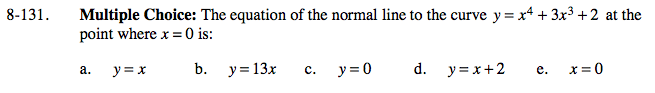### Home > CALC > Chapter 8 > Lesson 8.3.3 > Problem8-131

8-131.
1. Multiple Choice: The equation of the normal line to the curve y = x4 + 3x3 + 2 at the point where x = 0 is: Homework Help ✎

1. y = x

2. y = 13x

3. y = 0

4. y = x + 2

5. x = 0A 'Normal line' is perpendicular to a 'tangent line' at the point of tangency. So first find the equation of the tangent line, then manipulate its slope.